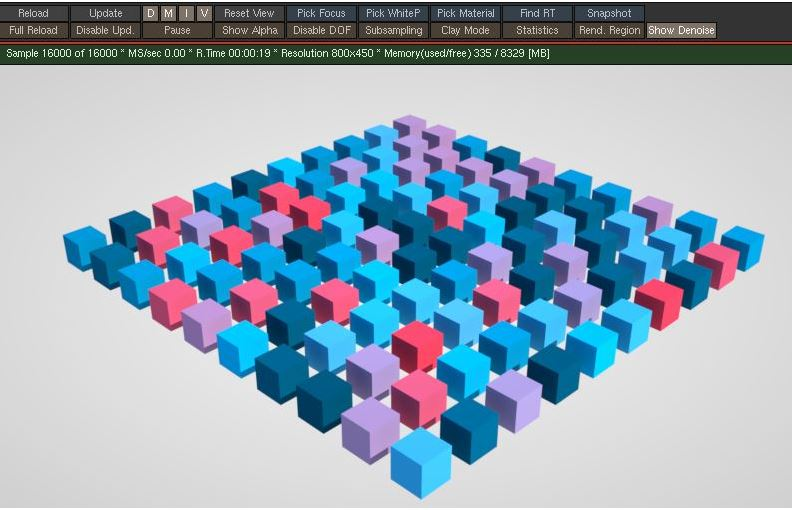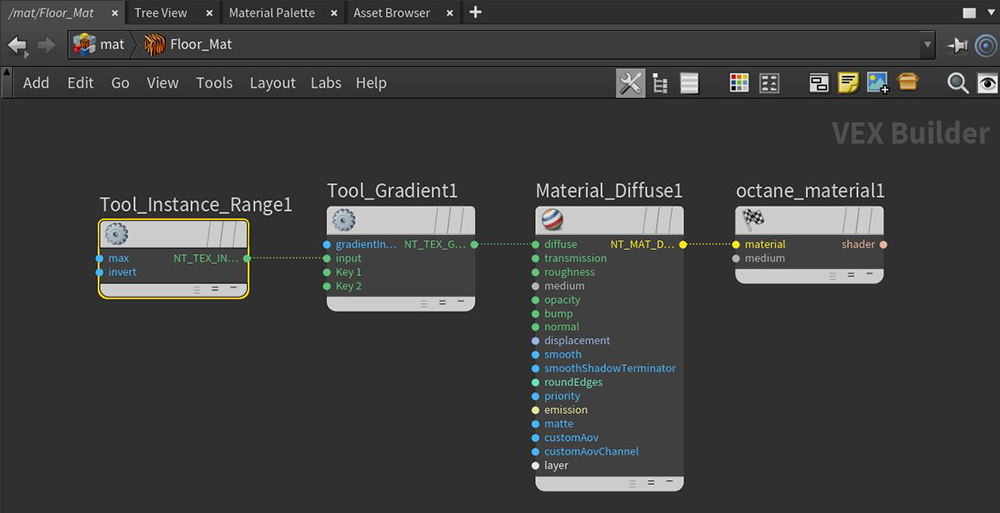Tools Instance Range

The Instance Range tool holds a gradient color with the range of 0 - Maximum ID, and prepares this map this range to geometric instance IDs (Figure 1). This node has one attribute, Maximum ID, which should correspond to the applied material's total number of instanced geometry in the scene.Figure 1: The Instance Range texture maps a gradient onto a series of instanced cubes

Figure 2 illustrates the typical shading network for utilizing the Instance Range node.Figure 2: Using the Instance Range node with a Gradient